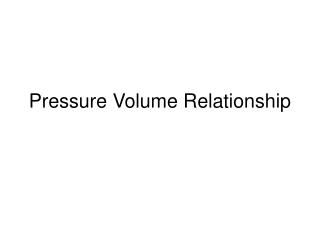DownloadDownload PresentationPressure Volume Relationship

# Pressure Volume Relationship

Télécharger la présentation## Pressure Volume Relationship

- - - - - - - - - - - - - - - - - - - - - - - - - - - E N D - - - - - - - - - - - - - - - - - - - - - - - - - - -
##### Presentation Transcript

1. Pressure Volume Relationship

2. Objectives • Explain Boyle's law. • Define pressure in general terms. • Compare atmospheric, hydrostatic pressure, and absolute pressure. • Correctly calculate absolute pressure for any depth of fresh or salt water • Correctly calculate pressure/volume relationships for a gas in a flexible container at any depth of fresh or salt water. • Correctly calculate the volume of gas in a cylinder of known volume, given a change in pressure.

3. Boyle’s Law: • Volume varies inversely with absolute pressure. • Density varies directly with absolute pressure.

4. Pressure • Definition • Force per unit area. • Atmospheric Pressure • Force exerted by the air column above us. • 14.7 psi at sea level = 1 atm. • Hydrostatic Pressure • Force exerted by the water column above us. • Every 33' of sw = 1atm. (increase per foot of depth = .445 psi) • Every 34' of fw = 1 atm. (increase per foot of depth = .432 psi) • Absolute Pressure • Sum of atmospheric and hydrostatic pressure. • Can be expressed as • Atmospheres absolute (ATA) • Psi absolute (PSIA)

5. Example 1 • Calculate the Absolute Pressure, in psia, at 61 feet in the ocean. Answer: Hydrostatic Pressure = .445psi/ft x 61 ft = 27.14 psi Atmospheric Pressure = 14.7 psi Absolute Pressure = 41.84psia

6. Example 2 Calculate the Absolute Pressure at 99' in the ocean in terms of ATA. Method 1 Use the formula (Depth + 33)/33 Depth = (99+33)/33 = 132/33 = 4ATA Method 2 Use the formula (Depth/33) + 1 = Depth ATA Depth ata = (99/33) + 1 = 3atm +1atm = 4ata Method 3 99 x .445psi = 44.055psi + 14.7psi = 58.7psi/14.7psi= 4ATA

7. Pressure/Volume Relationship • Formula • P1 x V1 = P2 x V2 • P1 = Original Pressure • V1 = Original Volume • P2 = New Pressure • V2 = New Volume

8. Example 1 A BC has 6 quarts of air at the surface and is submerged to 49' of fresh water. What will the volume of air be at this depth? Answer: P1 = Pressure at the surface = 1atm = 14.7psi V1 = Volume at the surface given as = 6 quarts P2= 49ffw x .432psi/ffw = 21.162psi + 14.7psi = 35.9psia V2 = ?

9. Example 1 continued Using P1 V1 = P2 V2 14.7psi x 6 quarts = 35.9 psia x V2 (14.7psi x 6 quarts) / 35.9psia = V2 88.2/35.9 = V2 2.45 quarts = V2

10. Example 2 A BC has 6 quarts of air at the surface and is submerged to 49 ffw. What will the volume of air be at this depth? P1 = Pressure at the surface = 1atm V1 = Volume at the surface given as = 6 quarts P2= (49ffw +34ffw) / 34ffw = 2.44 atm V2 = ?

11. Example 2 Continued Using P1 V1 = P2 V2 1 atm x 6 quarts = 2.44 atm x V2 (1 atm x 6 quarts) / 2.44 atm = V2 6 / 2.44 = V2 2.46 quarts = V2

12. Volume of air in a cylinder The volume of air in a cylinder is determined by the pressure of the gas in the cylinder and the internal volume of the cylinder. As the pressure in the cylinder drops, the volume of gas decreases. When you know the working pressure and volume of a cylinder, you can calculate the volume for any given pressure. This is done using the formula P1 / V1 = P2 / V2. Calculations require that we cross multiply and divide However, you must convert P1 and P2 to absolute pressure by adding 14.7 psi.

13. Example 1 A cylinder contains 50 cubic feet of air at a pressure of 3000psig. If the temperature remain constant, approximately how many cubic feet of air remain in the cylinder at 2000psig P1 = 3000 psig + 14.7 psi =3014.7 psia V1 = 50 cu. ft. P2 = 2000 psig + 14.7 psi = 2014.7 psia V2 = ?

14. Example 1 continued Use P1/V1 = P2 /V2 (remember to cross multiply and divide) 3014.7 psia = 2014.7 psia 50 cu. ft. V2 3014.7 psia x V2 = 2014.7 psia x 50 cu. Ft. 3014.7 psia x V2 = 100735 V2 = 100735/3014.7 V2 = 33.4 cu. ft.